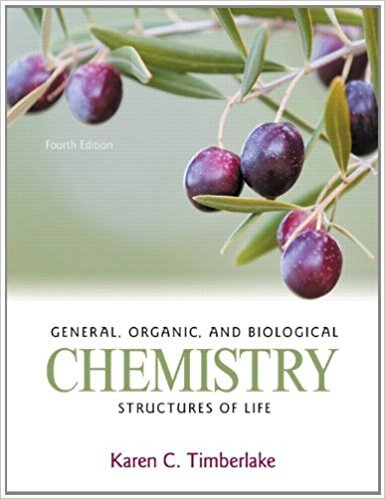×
Get Full Access to General, Organic, And Biological Chemistry: Structures Of Life - 4 Edition - Chapter 6 - Problem 119cq
Get Full Access to General, Organic, And Biological Chemistry: Structures Of Life - 4 Edition - Chapter 6 - Problem 119cq

×

# When 12.8 g of Na and 10.2 g of Cl2 react, what is theISBN: 9780321750891 35

## Solution for problem 119CQ Chapter 6

General, Organic, and Biological Chemistry: Structures of Life | 4th Edition

• Textbook Solutions
• 2901 Step-by-step solutions solved by professors and subject experts
• Get 24/7 help from StudySoup virtual teaching assistantsGeneral, Organic, and Biological Chemistry: Structures of Life | 4th Edition

4 5 1 406 Reviews
11
0
Problem 119CQ

When 12.8 g of Na and 10.2 g of Cl2 react, what is the mass, in grams, of NaCl that is produced?2Na(s) + Cl2(g) ? 2NaCl(s)

Step-by-Step Solution:

Solution 119CQStep 1 of 3:Here, we are asked to find the grams of NaCl produced.Given reaction:2Na(s) + Cl2(g) 2NaCl(s)Given:Mass of Na = 12.8 g Mass of Cl2 = 10.2 g Grams of NaCl =Molar mass of Na = 22.989 g/molMolar mass of S = 70.906 g/molFirst, let’s write the mole-mole factors for the above reaction:2 mol of Na = 2 mol of NaCl. So, the mole-mole factor is: and 1 mol of Cl2 = 2 mol of NaCl. So, the mole-mole factor is: and The mole-mass factor of Na is:1 mol Na = 22.989 g Na. So, the mole-mass factor isand The mole-mass factor of Cl2 is:1 mol Cl2 = 70.906 g. So, the mole-mass factor isand ________________

Step 2 of 3

Step 3 of 3

##### ISBN: 9780321750891

This textbook survival guide was created for the textbook: General, Organic, and Biological Chemistry: Structures of Life, edition: 4. The answer to “When 12.8 g of Na and 10.2 g of Cl2 react, what is the mass, in grams, of NaCl that is produced?2Na(s) + Cl2(g) ? 2NaCl(s)” is broken down into a number of easy to follow steps, and 26 words. The full step-by-step solution to problem: 119CQ from chapter: 6 was answered by , our top Chemistry solution expert on 05/06/17, 06:54PM. Since the solution to 119CQ from 6 chapter was answered, more than 693 students have viewed the full step-by-step answer. This full solution covers the following key subjects: . This expansive textbook survival guide covers 24 chapters, and 2445 solutions. General, Organic, and Biological Chemistry: Structures of Life was written by and is associated to the ISBN: 9780321750891.

Unlock Textbook Solution